QuestionChapter 3, Problem 1R
Program Plan Intro

## Pseudorandom Number generation:In Java, there is a built-in class java.util.Random whose object as pseudorandom number to determine the sequence of numbers by randomly.Note: Refer page number 113 to formula for next pseudorandom number in the text book.According the formula given by the text book, the current seed “cur” value is needed to find the next pseudorandom number. But, here the only one current seed “cur” is given to find the five next pseudorandom numbers. Apply the given inputs for five times; get the same next pseudorandom number. So, take current seed value as “next pseudorandom number” to find the next pseudorandom numbers.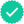### Explanation of Solution

Determine the next five pseudorandom numbers:

The given inputs are,

a = 12

b = 5

n = 100

Current seed (cur) = 92

The formula for next pseudorandom number is given below:

next = (a × cur + b)% n        (1)

First pseudorandom numbers:

Substitute the “a”, “b”, “n”, and “cur” in the Equation (1) to determine the first next pseudorandom number is given below:

next = (12 × 92 + 5)% 100(1104 + 5)% 100(1109)% 100= 9

Therefore, the first pseudorandom number for current seed (cur = 92) is 9.

Second pseudorandom numbers:

Here, let us consider the current seed “cur” as “9”. That is, result of first pseudorandom number.

Substitute the “a”, “b”, “n”, and “cur” in the Equation (1) to determine the first next pseudorandom number is given below:

next = (12 × 9 + 5)% 100(108 + 5)% 100(113)% 100= 13

Therefore, the second pseudorandom number for current seed (cur = 9) is 13.

Third pseudorandom numbers:

Here, let us consider the current seed “cur” as “13”. That is, result of second pseudorandom number.

Substitute the “a”, “b”, “n”, and “cur” in the Equation (1) to determine the first next pseudorandom number is given below:

next = (12 × 13 + 5)% 100(156 + 5)% 100(161)% 100= 61

Therefore, the third pseudorandom number for current seed (cur = 13) is 61.

Fourth pseudorandom numbers:

Here, let us consider the current seed “cur” as “61”. That is, result of second pseudorandom number.

Substitute the “a”, “b”, “n”, and “cur” in the Equation (1) to determine the first next pseudorandom number is given below:

next = (12 × 61 + 5)% 100(732 + 5)% 100(737)% 100= 37

Therefore, the fourth pseudorandom number for current seed (cur = 61) is 37.

Fifth pseudorandom numbers:

Here, let us consider the current seed “cur” as “37”. That is, result of second pseudorandom number.

Substitute the “a”, “b”, “n”, and “cur” in the Equation (1) to determine the first next pseudorandom number is given below:

next = (12 × 37 + 5)% 100(444 + 5)% 100(449)% 100= 49

Therefore, the fourth pseudorandom number for current seed (cur = 37) is 49.

### Want to see more full solutions like this?

Subscribe now to access step-by-step solutions to millions of textbook problems written by subject matter experts!
03:09
Students have asked these similar questions
Trace the following pieces of code and give their exact output and the tracing steps.
Give the steps to eliminate useless symbols?
We can express an arithmetic shift in a few sentences.
Knowledge BoosterRecommended textbooks for you
•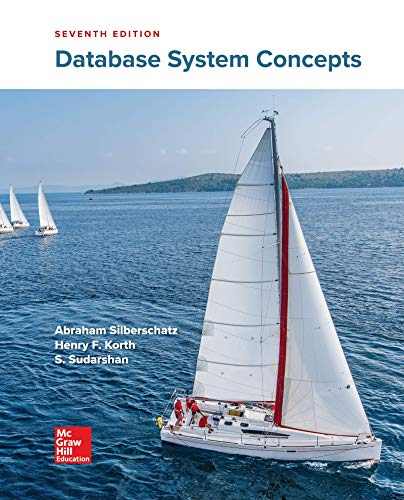Database System Concepts
Computer Science
ISBN:9780078022159
Author:Abraham Silberschatz Professor, Henry F. Korth, S. Sudarshan
Publisher:McGraw-Hill Education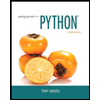Starting Out with Python (4th Edition)
Computer Science
ISBN:9780134444321
Publisher:PEARSONDigital Fundamentals (11th Edition)
Computer Science
ISBN:9780132737968
Author:Thomas L. Floyd
Publisher:PEARSON
•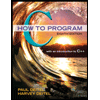C How to Program (8th Edition)
Computer Science
ISBN:9780133976892
Author:Paul J. Deitel, Harvey Deitel
Publisher:PEARSONDatabase Systems: Design, Implementation, & Manag...
Computer Science
ISBN:9781337627900
Author:Carlos Coronel, Steven Morris
Publisher:Cengage Learning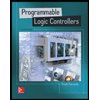Programmable Logic Controllers
Computer Science
ISBN:9780073373843
Author:Frank D. Petruzella
Publisher:McGraw-Hill Education
•Database System Concepts
Computer Science
ISBN:9780078022159
Author:Abraham Silberschatz Professor, Henry F. Korth, S. Sudarshan
Publisher:McGraw-Hill EducationStarting Out with Python (4th Edition)
Computer Science
ISBN:9780134444321
Publisher:PEARSONDigital Fundamentals (11th Edition)
Computer Science
ISBN:9780132737968
Author:Thomas L. Floyd
Publisher:PEARSONC How to Program (8th Edition)
Computer Science
ISBN:9780133976892
Author:Paul J. Deitel, Harvey Deitel
Publisher:PEARSONDatabase Systems: Design, Implementation, & Manag...
Computer Science
ISBN:9781337627900
Author:Carlos Coronel, Steven Morris
Publisher:Cengage LearningProgrammable Logic Controllers
Computer Science
ISBN:9780073373843
Author:Frank D. Petruzella
Publisher:McGraw-Hill Education